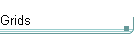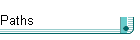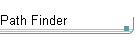## Partial list of path functions

Path functions are needed as you need to generate a path ID to pass to the mp_grid_path function. Also you will need to extract details of the path once it has been generated.

This list omits the functions relating to basic path movementpath_add() This function generates an empty path and returns its ID. You would typically generate an empty path in the create event of the instance that will use the pathpath_delete(index) This function disposes of a path to free up the memory used If you created the empty path in the create event you might delete the path in the destroy eventpath_get_number(index) This function returns the number of POINTS that make up the path, the points are numbered from 0 to n-1.path_get_point_x(index, n)path_get_point_y(index, n) These functions return the x,y coordinates of a point on the path. If you want to use the path as a navigation guide rather than a strict path then you will need to extract the coordinates and feed them into your "A.I.".path_delete_point(index, n) This function deletes point n of the path. Note that if you are looping through the points the numbers of the following points will be moved down by one after deletion, so if you are looping through the points deleting unwanted ones then it may be preferable to count down not up.